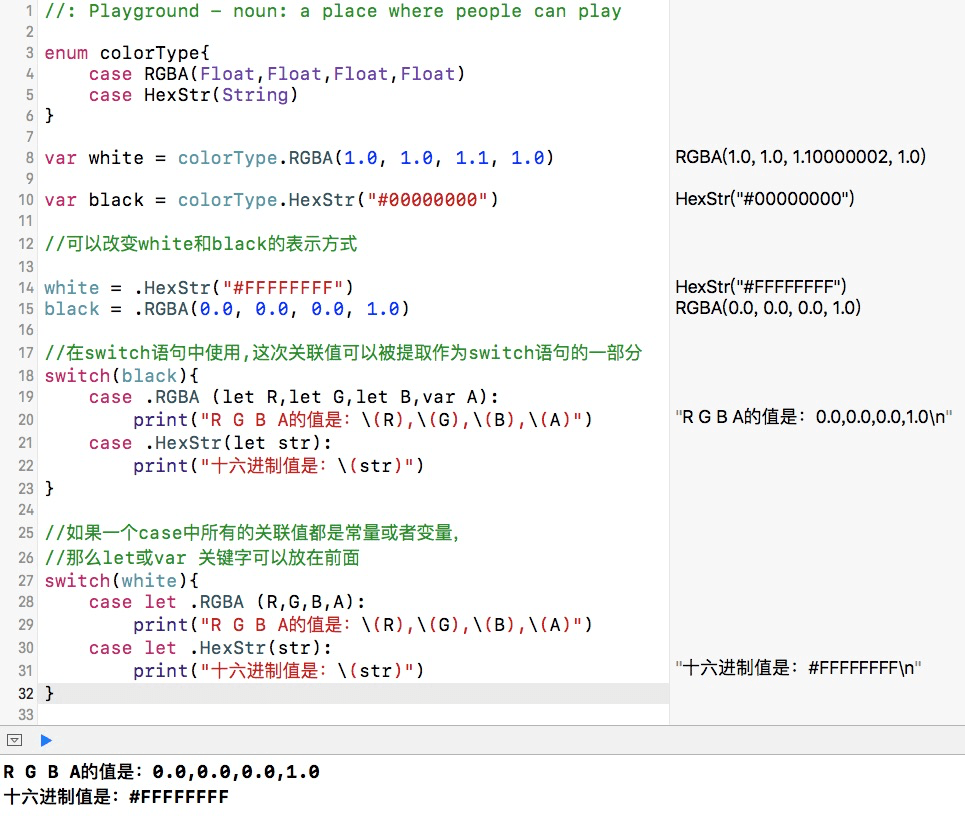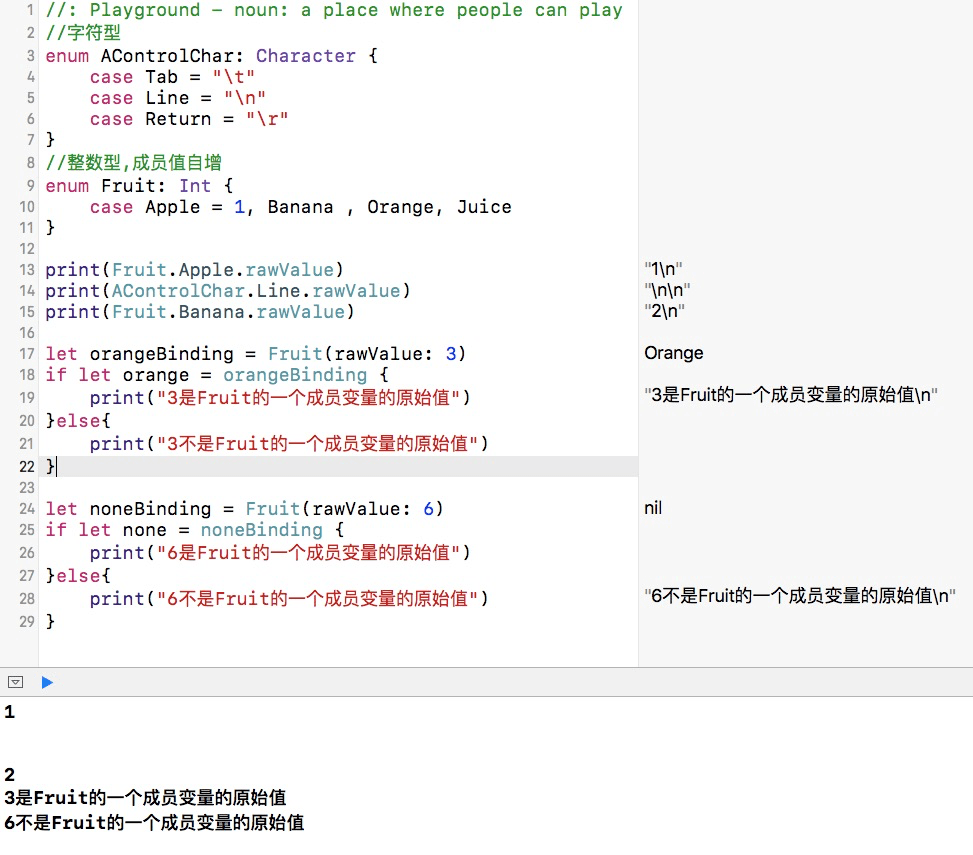# 枚举语法

``````enum colorElement {
case R
case G
case B
case A
}
//或者直接逗号分隔进行定义
enum colorElementToo{
case R, G, B, A
}
var colorE = colorElement.R
//知道colorE的类型后，下次赋值时可以省略枚举类型名
colorE = colorElement.G

switch colorE {
case .R:
print("红")
case .G:
print("绿")
case .B:
print("蓝")
case .A:
print("透明")
}
//打印结果：绿
``````

# 关联值

``````enum colorType{
case RGBA(Float,Float,Float,Float)
case HexStr(String)
}

var white = colorType.RGBA(1.0, 1.0, 1.1, 1.0)

var black = colorType.HexStr("#00000000")

//可以改变white和black的表示方式

white = .HexStr("#FFFFFFFF")
black = .RGBA(0.0, 0.0, 0.0, 1.0)

//在switch语句中使用,这次关联值可以被提取作为switch语句的一部分
switch(black){
case .RGBA (let R,let G,let B,var A):
print("R G B A的值是：\(R),\(G),\(B),\(A)")
case .HexStr(let str):
print("十六进制值是：\(str)")
}

//如果一个case中所有的关联值都是常量或者变量，
//那么let或var 关键字可以放在前面
switch(white){
case let .RGBA (R,G,B,A):
print("R G B A的值是：\(R),\(G),\(B),\(A)")
case let .HexStr(str):
print("十六进制值是：\(str)")
}
``````# 原始值

``````//字符型
enum AControlChar: Character {
case Tab = "\t"
case Line = "\n"
case Return = "\r"
}
//整数型,成员值自增
enum Fruit: Int {
case Apple = 1, Banana , Orange, Juice
}
// Banana的原始值是2, Orange的原始值是3
``````

``````//字符型
enum AControlChar: Character {
case Tab = "\t"
case Line = "\n"
case Return = "\r"
}
//整数型,成员值自增
enum Fruit: Int {
case Apple = 1, Banana , Orange, Juice
}

print(Fruit.Apple.rawValue)
print(AControlChar.Line.rawValue)
print(Fruit.Banana.rawValue)

let orangeBinding = Fruit(rawValue: 3)
if let orange = orangeBinding {
print("3是Fruit的一个成员变量的原始值")
}else{
print("3不是Fruit的一个成员变量的原始值")
}

let noneBinding = Fruit(rawValue: 6)
if let none = noneBinding {
print("6是Fruit的一个成员变量的原始值")
}else{
print("6不是Fruit的一个成员变量的原始值")
}
``````# 示例代码

https://github.com/99ios/23.10